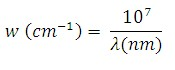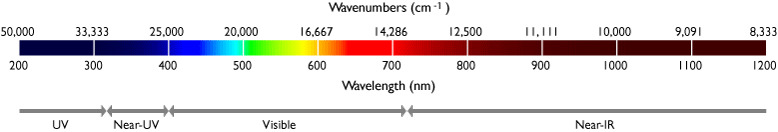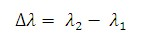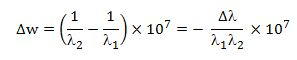Switch Customer
• ##### {{category.Description}}View All Resources

# Measuring Light with Wavelengths and Wavenumbers

The "color" of light is generally identified by the distribution of power or intensity as a function of wavelength λ.  Visible light has a wavelength that ranges from about 400 nm to just over 700 nm.  Sometimes it is convenient to describe light in terms of units called "wavenumbers," where the wavenumber w is typically measured in units of cm-1 ("inverse centimeters") and is simply equal to the inverse of the wavelength, and is therefore proportional to frequency:In applications like Raman spectroscopy, both wavelength and wavenumber units are often used together, leading to potential confusion.  The laser lines are generally identified by wavelength, but the separation of a particular Raman line from the laser line is generally given by a "wavenumber shift" Δw, since the frequency of the response is fixed by the molecular properties of the material and independent of which laser wavelength is used to excite the line.

When speaking of a "shift" from a first known wavelength λ1 to a second known wavelength λ2, the resulting wavelength shift Δλ is given bywhereas the resulting wavenumber shift Δw is given byWhen speaking of a known wavenumber shift Δw from a first known wavelength λ1, the resulting second wavelength λ2 is given byNote that when the final wavelength λ2 is longer than the initial wavelength λ1, which corresponds to a "red shift," in the above equations Δw < 0, consistent with a shift toward smaller values of w. However, when the final wavelength λ2 is shorter than the initial wavelength λ1, which corresponds to a "blue shift," Δw > 0, consistent with a shift toward larger values of w.